Quantity: 0

Total: 0,00

0

# Volume of a tetrahedron

### Related extras#### Plotting a perpendicular to a line at a given point P on the line

Both line e and point P, which is situated on it, are given. Let’s plot line g to include point...#### Sphere

A sphere is the set of points which are all within the same distance from a given point...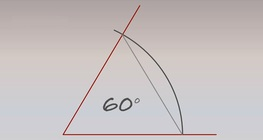#### Plotting a 60° angle

To plot a 60° angle, we refer you back to the plotting of an equilateral triangle.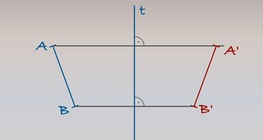#### Reflecting a line segment across an axis

Axis t and line segment AB are given on a plane. Let’s plot the mirror image of line segment AB...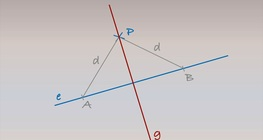#### Plotting a perpendicular on a line from a point P that is not on the line

Both line e and point P, which is not situated on line e, are given. Let’s plot line g such that...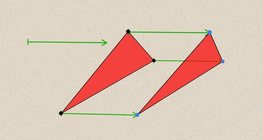#### Geometric transformations – translation

This animation demonstrates geometric translation in both plane and space.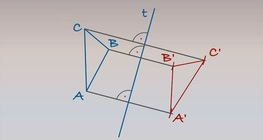#### Reflecting a triangle across an axis

Axis t and triangle ABC are given on a particular plane. Let’s plot the mirror image of triangle...#### Cube of cubes

An exercise about the regular hexahedron built from unit cubes to help deepen your...

Added to your cart.